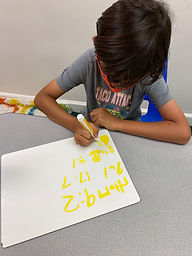## Ms. Kylie

### Target 1​

###### Lesson Type:

Continuation

Data Probability

:

Ratio

Understand ratio concepts and use ratio reasoning to solve problems.

###### 1:

Understand that a ratio represents a comparison of two quantities.

6th

###### Vocabulary:

Ratio

Activities:

• Students compared the quantity of two things around the room to the find the ratio.
• Students were given story problems and wrote the ratio of the two things being compared.### Home Exploration

###### Guiding Questions:## Absent Students:

### Target 2

:

###### 1:

Identify the relating factor in an analogy.

###### 2:

Identify the target and analogue concept.

5th

###### Vocabulary:

Analogy, Relating Factor

Activities:

• Students studied the given analogy statements to find the relating factors.### Home Exploration

###### Guiding Questions:### Target 3

:

###### 1:

Understand that an exponent indicated the number of times that a base is multiplied by itself.

###### 2:

Write the expanded format for a given exponent (repeating multiplication equation that is equivalent to a given exponent).

###### 3:

Write an exponent that is equivalent to the expanded format (a repeating multiplication equation).

###### 4:

Evaluate expressions containing positive exponents.

6th

###### Vocabulary:

Exponent, Base, Power, Expanded Notation, Standard Notation

Activities:

• Students learned exponents are a shortened way to represent repeated multiplication of the same number.
• Students were given an exponent and broke down the exponent by writing the base number, exponent, expanded notation form and the standard notation form.
• Students solved different exponents.### Home Exploration

Evaluate the exponent.

• What is the number you are multiplying by itself?
• What number tells you how many times you are multiplying that number?
• Write the exponent in expanded notation.

64